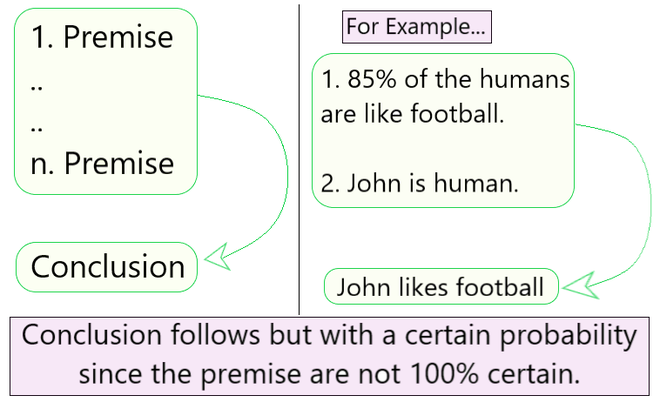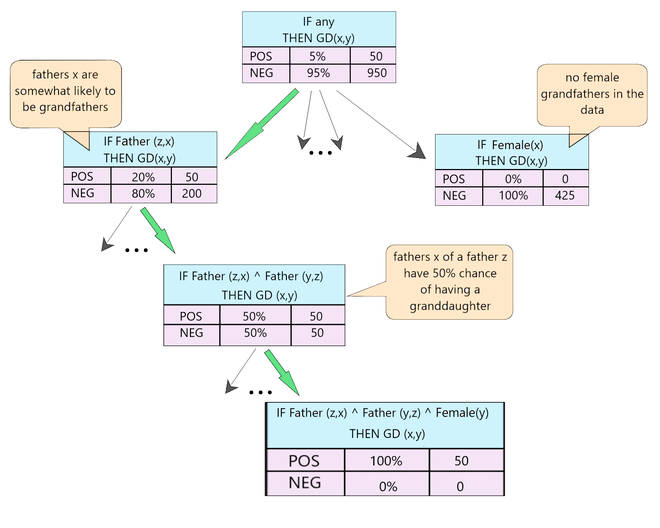Related Articles
First-Order Inductive Learner (FOIL) Algorithm
• Last Updated : 26 Nov, 2020

Before getting into the FOIL Algorithm, let us understand the meaning of first-order rules and the various terminologies involved in it.

First-Order Logic:

All expressions in first-order logic are composed of the following attributes:

1. constants — e.g. tyler, 23, a
2. variables — e.g. A, B, C
3. predicate symbols — e.g. male, father (True or False values only)
4. function symbols — e.g. age (can take on any constant as a value)
5. connectives — e.g. ∧, ∨, ¬, →, ←
6. quantifiers — e.g. ∀, ∃

Term: It can be defined as any constant, variable or function applied to any term. e.g. age(bob)

Literal: It can be defined as any predicate or negated predicate applied to any terms. e.g. female(sue), father(X, Y)

It has 3 types:

• Ground Literal — a literal that contains no variables. e.g. female(sue)
• Positive Literal — a literal that does not contain a negated predicate. e.g. female(sue)
• Negative Literal — a literal that contains a negated predicate. e.g. father(X,Y)

Clause – It can be defined as any disjunction of literals whose variables are universally quantified.where,

M1, M2, ...,Mn --> literals (with variables universally quantified)
V --> Disjunction (logical OR)

Horn clause — It can be defined as any clause containing exactly one positive literal.Since,andThen the horn clause can be written aswhere,
H --> Horn Clause
L1,L2,...,Ln --> Literals

(A ⇠ B) --> can be read as 'if B then A' [Inductive Logic]

and

∧ --> Conjunction (logical AND)
∨ --> Disjunction (logical OR)
¬ --> Logical NOT

First Order Inductive Learner (FOIL)

In machine learning, first-order inductive learner (FOIL) is a rule-based learning algorithm. It is a natural extension of SEQUENTIAL-COVERING and LEARN-ONE-RULE algorithms. It follows a Greedy approach.

Inductive Learning:

Inductive learning analyzing and understanding the evidence and then using it to determine the outcome. It is based on Inductive Logic.Fig 1: Inductive Logic

Algorithm Involved

FOIL(Target predicate, predicates, examples)

• Pos ← positive examples
• Neg ← negative examples
• Learned rules ← {}
• while Pos, do
//Learn a NewRule
– NewRule ← the rule that predicts target-predicate with no preconditions

– NewRuleNeg ← Neg
– while NewRuleNeg, do
Add a new literal to specialize NewRule
1. Candidate_literals ← generate candidates for newRule based on Predicates
2. Best_literal ←
argmaxL∈Candidate literalsFoil_Gain(L,NewRule)

3. add Best_literal to NewRule preconditions
4. NewRuleNeg ← subset of NewRuleNeg that satisfies NewRule preconditions
– Learned rules ← Learned rules + NewRule
– Pos ← Pos − {members of Pos covered by NewRule}

• Return Learned rules


Working of the Algorithm:

In the algorithm, the inner loop is used to generate a new best rule. Let us consider an example and understand the step-by-step working of the algorithm.Fig 2 : FOIL Example

Say we are tying to predict the Target-predicate- GrandDaughter(x,y).
We perform the following steps: [Refer Fig 2]

Step 1 - NewRule = GrandDaughter(x,y)
Step 2 -
2.a - Generate the candidate_literals.
(Female(x), Female(y), Father(x,y), Father(y.x),
Father(x,z), Father(z,x), Father(y,z), Father(z,y))

2.b - Generate the respective candidate literal negations.
(¬Female(x), ¬Female(y), ¬Father(x,y), ¬Father(y.x),
¬Father(x,z), ¬Father(z,x), ¬Father(y,z), ¬Father(z,y))

Step 3 - FOIL might greedily select Father(x,y) as most promising, then
NewRule = GrandDaughter(x,y) ← Father(y,z) [Greedy approach]

Step 4 - Foil now considers all the literals from the previous step as well as:
(Female(z), Father(z,w), Father(w,z), etc.) and their negations.

Step 5 - Foil might select Father(z,x), and on the next step Female(y) leading to
NewRule = GrandDaughter (x,y) ← Father(y,z) ∧ Father(z,x) ∧ Female(y)

Step 6 - If this greedy approach covers only positive examples it terminates
the search for further better results.

FOIL now removes all positive examples covered by this new rule.
If more are left then the outer while loop continues.


FOIL: Performance Evaluation Measure

The performance of a new rule is not defined by its entropy measure (like the PERFORMANCE method in Learn-One-Rule algorithm).

FOIL uses a gain algorithm to determine which new specialized rule to opt. Each rule’s utility is estimated by the number of bits required to encode all the positive bindings. [Eq.1]where,
L is the candidate literal to add to rule R

p0 = number of positive bindings of R
n0 = number of negative bindings of R
p1 = number of positive binding of R + L
n1 = number of negative bindings of R + L
t  = number of positive bindings of R also covered by R + L


FOIL Algorithm is another rule-based learning algorithm that extends on the Sequential Covering + Learn-One-Rule algorithms and uses a different Performance metrics (other than entropy/information gain) to determine the best rule possible. For any doubts/queries regarding the algorithm, comment below.My Personal Notes arrow_drop_up